# Complete Symmetric Figures (2)

In this worksheet, students must complete shapes so that they are symmetrical in the given slanting line of symmetry.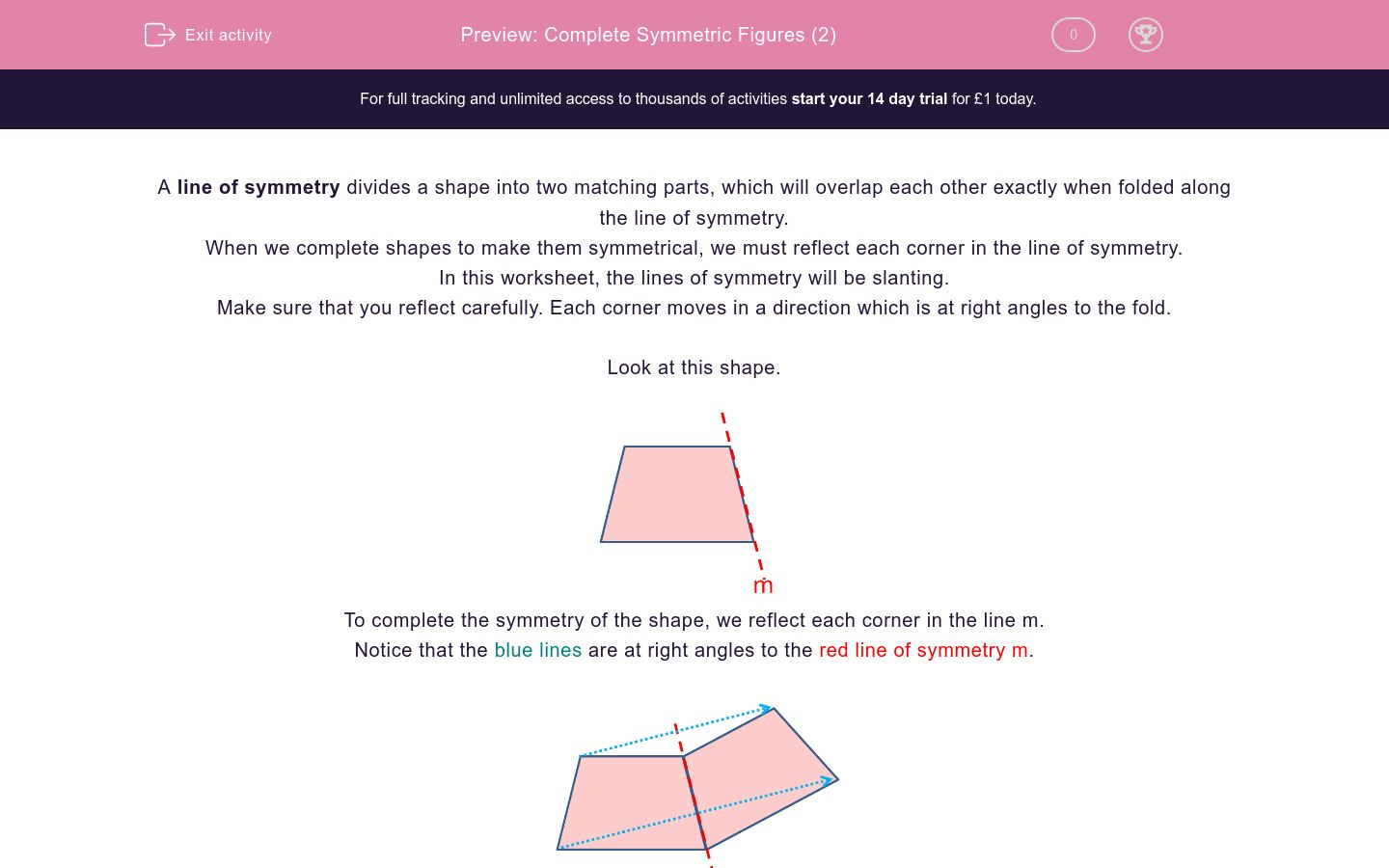Key stage:  KS 2

Curriculum topic:   Maths and Numerical Reasoning

Curriculum subtopic:   Symmetry

Difficulty level:### QUESTION 1 of 10

A line of symmetry divides a shape into two matching parts, which will overlap each other exactly when folded along the line of symmetry.

When we complete shapes to make them symmetrical, we must reflect each corner in the line of symmetry.

In this worksheet, the lines of symmetry will be slanting.

Make sure that you reflect carefully. Each corner moves in a direction which is at right angles to the fold.

Look at this shape.To complete the symmetry of the shape, we reflect each corner in the line m.

Notice that the blue lines are at right angles to the red line of symmetry m.The next shape has been completed WRONGLY.

Notice that the blue lines are NOT AT RIGHT ANGLES to the red line of symmetry m.This is how it should be completed.

Notice that the blue lines are at right angles to the red line of symmetry m.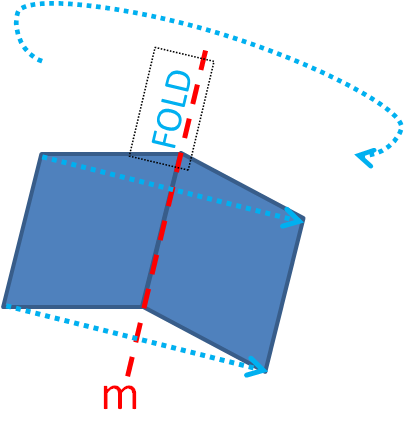Look at this shape.Which diagram shows the completed symmetrical shape?A

B

Look at this shape.Which diagram shows the completed symmetrical shape?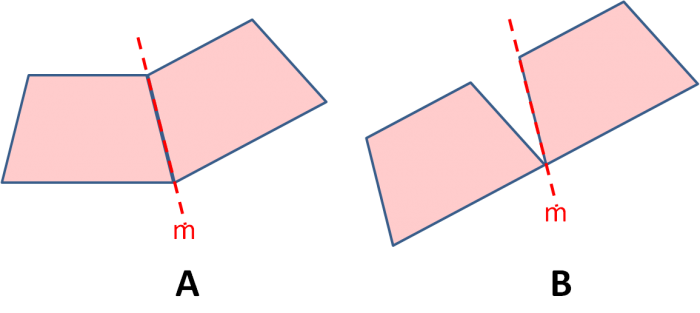A

B

Look at this shape.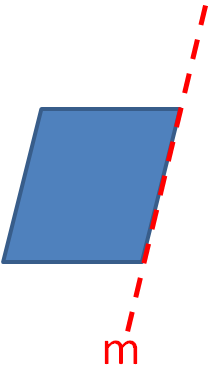Which diagram shows the completed symmetrical shape?A

B

Look at this shape.Which diagram shows the completed symmetrical shape?A

B

Look at this shape.Which diagram shows the completed symmetrical shape?A

B

Look at this shape.Which diagram shows the completed symmetrical shape?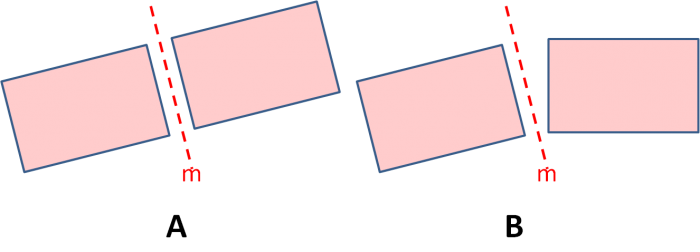A

B

Look at this shape.Which diagram shows the completed symmetrical shape?A

B

Look at this shape.Which diagram shows the completed symmetrical shape?A

B

Look at this shape.Which diagram shows the completed symmetrical shape?A

B

Look at this shape.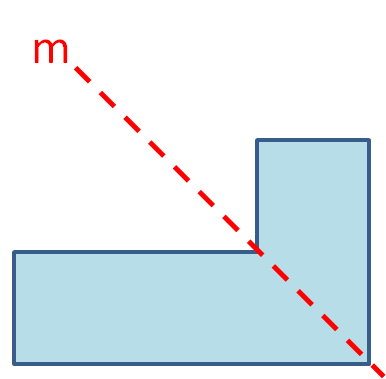Which diagram shows the completed symmetrical shape?A

B

• Question 1

Look at this shape.Which diagram shows the completed symmetrical shape?A
EDDIE SAYS
There is no gap between the mirror line and the rectangle.
• Question 2

Look at this shape.Which diagram shows the completed symmetrical shape?A
EDDIE SAYS
There is no gap between the mirror line and the trapezium.
• Question 3

Look at this shape.Which diagram shows the completed symmetrical shape?A
EDDIE SAYS
The direction of the fold must be at right angles to the mirror line.
• Question 4

Look at this shape.Which diagram shows the completed symmetrical shape?B
EDDIE SAYS
The two short sides match and the two long sides match across the mirror line.
• Question 5

Look at this shape.Which diagram shows the completed symmetrical shape?B
EDDIE SAYS
• Question 6

Look at this shape.Which diagram shows the completed symmetrical shape?A
EDDIE SAYS
The distance between the two shapes and the mirror line must be equal all along the length.
• Question 7

Look at this shape.Which diagram shows the completed symmetrical shape?B
EDDIE SAYS
Shape B is symmetrical whereas Shape A is not.
• Question 8

Look at this shape.Which diagram shows the completed symmetrical shape?A
EDDIE SAYS
Shape A is symmetrical whereas Shape B is not.
• Question 9

Look at this shape.Which diagram shows the completed symmetrical shape?A
EDDIE SAYS
Shape A is symmetrical whereas Shape B is not.
• Question 10

Look at this shape.Which diagram shows the completed symmetrical shape?B
EDDIE SAYS
Shape B is symmetrical and overlaps the original shape, because the line of symmetry actually goes through the original shape.
---- OR ----

Sign up for a £1 trial so you can track and measure your child's progress on this activity.

### What is EdPlace?

We're your National Curriculum aligned online education content provider helping each child succeed in English, maths and science from year 1 to GCSE. With an EdPlace account you’ll be able to track and measure progress, helping each child achieve their best. We build confidence and attainment by personalising each child’s learning at a level that suits them.

Get started